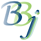# BBj Object Error Handling

## Description

BBj allows object expressions to be used anywhere in the language. It does not attempt to do type checking until runtime. For example, assuming that NUM!=123 and STR!="123", all of the following statements are accepted as valid syntax, but all generate errors at runtime:

 N = NUM!+STR!; REM ' NUM+STR is an undefined operation I = Integer.parseInt(NUM!); REM ' no such method I! = new Integer(STR!); REM ' no such method

The STR() function can be used to convert a numeric or string object expression to a string. For example:

 S\$ = STR(NUM!) + STR(STR!); REM S\$ = "123" + "123" = "123123"

Similarly, the NUM() function can be used to convert a numeric or string object expression to a number. For example:

 N = NUM(NUM!) + NUM(STR!); REM N = 123 + 123 = 246

The ERR= option can be used in the NUM() function if there is any chance that a conversion error might occur. The STR() function will never generate a conversion error, but it also allows the ERR= option for syntactic completeness.

The ERR= option can also be used to trap errors from Java calls. For example, the following code fragment will loop until the user enters a valid binary number:

 0010 loop: INPUT "Enter a binary value: ",binary\$ 0020 decimal = Integer.parseInt(binary\$,2,ERR=loop) 0030 PRINT "Binary ",binary\$," is decimal",decimal >run Enter a binary value: 123 Enter a binary value: 100 Binary 100 is decimal 4

The ERR= option is allowed at the end of the argument list of any Java constructor or method call. Some examples are:

 0010 h! = new java.util.HashMap(ERR=*NEXT) 0020 i! = new java.util.ArrayList(n,ERR=*NEXT) 0030 INPUT "float?",f\$; LET f = Float.parseFloat(f\$,ERR=*SAME) >RUN float?33.33- float?-33.33

If embedded Java code throws an exception, BBj will catch that exception and will generate !ERROR=252. The exception information can be retrieved with:

E! is a java.lang.Throwable object; see the Java documentation for details.